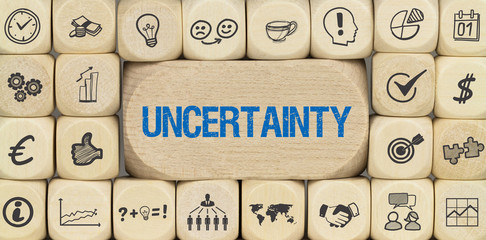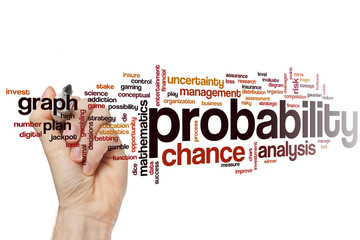# ProbabilityProbability is the language of chance.
There is chance of raining today. What is the chance that India will win the match? In this way lots more statements are used by us in our daily lives wherein we talk about chance.
To represent these “chance statements”, we have a section in mathematics known as Probability. This Probability concept is used in game of chance (cards/lotteries etc.), insurance sector, weather forecasting and a lot more.

Now the language of chance or Probability is expressed as fraction/decimal/percentage.
Probability is again the basis of Inferential Statistics. Descriptive statistics gives us data and from that data if we make any prediction that is Inferential Statistics. Probability is again the science of prediction.

The language of chance or Probability can be found by three methods.
1.Experimental Probability or Empirical Probability or Relative Frequency Probability:
This is based on direct observation or experiment. Experiment means random experiment. Random means that can be repeated under same conditions.
Two things are important here –

•      More than one outcome
•      Not sure about outcome (during trials).

Trials means simply to try. Like toss of a coin, rolling a dice etc. We never know the outcome here. Again if there is only one outcome, there is no chance of doing an experiment. For example, the area of a circle is πr2. So, since only one outcome will come, what’s the importance of doing an experiment there.
Suppose we have to find the probability of getting prime numbers while rolling a dice. Here the event is “getting prime numbers when you roll a dice”. Suppose you and your cousin sit with the Ludo and wanna see whose prediction will come true!

So let’s try rolling a dice several times. We will record the observations in a Tally Chart.
First we will see the possible outcomes and write them in the first column. Here the possible outcomes are 6. Suppose while rolling the dice several times, 1 comes 2 times, 6 didn’t come, 2 comes 7 times, 3 comes 5 times, 4 comes 2 times and 5 comes 5 times.

17                        21 So in the second column note them in the form of tally and in the 3rd column write the frequencies of even numbers. Here frequency means number of times we get an even number. So, Probability is also called Relative Frequency Probability. Add the frequencies, this is the favorable number of times we will get our event that is prime numbers.
In the 4th column add the total number of times you rolled the dice. This is called as sample space n(S).
So now, come to formula-

That means probability is 17/21 or 0.8 or 80% (approx.)
2.Theoretical Probability.
This is fully based on mathematical analysis. No experiment is required. For example, to roll a dice and to find the probability of getting prime numbers. This is our event P(A) suppose. So, possible outcomes are (1,2,3,4,5,6). This is sample space n(S) suppose.
So, probability of finding prime numbers – That means, P (prime numbers) or –

Here n(A)=3 (because three prime numbers are there 2,3 and 5) and n(S)=6. This is theoretical probability. We will always get 1/2. But in experimental probability, the result may be different.

3.Subjective Probability
This is based on informed guesswork.
Suppose I have just come home from a 7 days holiday trip. How much is the probability that I will go on a trip the next day? Very less probability say …. 0.01 %.
I have bought a new pair of shoes and its number is 9. How much is the probability that I will buy the number 9 shoes next year? High probability say 90% as my feet size is not going to change next year.Advice for class 10 students! So, guys! in experimental probability we will do the experiment, record the findings in tally chart and then apply formula to find the probability.

Previous Article
Next Article